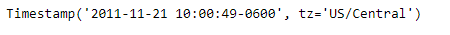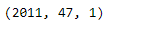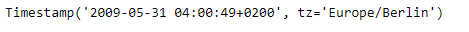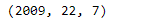Related Articles

# Python | Pandas Timestamp.isocalendar

• Last Updated : 14 Jan, 2019

Python is a great language for doing data analysis, primarily because of the fantastic ecosystem of data-centric python packages. Pandas is one of those packages and makes importing and analyzing data much easier.

Pandas` Timestamp.isocalendar()` function return a 3-tuple containing ISO year, week number, and weekday for the given Timestamp object.

Attention geek! Strengthen your foundations with the Python Programming Foundation Course and learn the basics.

To begin with, your interview preparations Enhance your Data Structures concepts with the Python DS Course. And to begin with your Machine Learning Journey, join the Machine Learning - Basic Level Course

Syntax :Timestamp.isocalendar()

Parameters : None

Return : Tuple

Example #1: Use `Timestamp.isocalendar()` function to return the date of the given Timestamp object based on ISO calendar.

 `# importing pandas as pd``import` `pandas as pd`` ` `# Create the Timestamp object``ts ``=` `pd.Timestamp(year ``=` `2011``,  month ``=` `11``, day ``=` `21``,``                  ``hour ``=` `10``, second ``=` `49``, tz ``=` `'US/Central'``)`` ` `# Print the Timestamp object``print``(ts)`

Output :Now we will use the `Timestamp.isocalendar()` function to return the date in ts object based on ISO calendar.

 `# return the date as an ISO calendar``ts.isocalendar()`

Output :As we can see in the output, the `Timestamp.isocalendar()` function has returned a tuple containing the year, week number and the week day for the given Timestamp object.

Example #2: Use `Timestamp.isocalendar()` function to return the date of the given Timestamp object based on ISO calendar.

 `# importing pandas as pd``import` `pandas as pd`` ` `# Create the Timestamp object``ts ``=` `pd.Timestamp(year ``=` `2009``, month ``=` `5``, day ``=` `31``,``                  ``hour ``=` `4``, second ``=` `49``, tz ``=` `'Europe/Berlin'``)`` ` `# Print the Timestamp object``print``(ts)`

Output :Now we will use the `Timestamp.isocalendar()` function to return the date in ts object based on ISO calendar

 `# return the date as an ISO calendar``ts.isocalendar()`

Output :As we can see in the output, the `Timestamp.isocalendar()` function has returned a tuple containing the year, week number and the week day for the given Timestamp object.

My Personal Notes arrow_drop_up